### 逻辑回归的算法介绍

• h函数： 根据输入的数据预测类别的函数，Andrew Ng的公开课中称为hypothesis function。
• j函数： 我们需要一个机制去评估我们的h函数的好坏,j函数的作用是评估h函数的好坏,一般这个函数称为损失函数(loss function)或者错误函数(error function)。

### 逻辑回归的数学原理

#### h函数相关（预测函数）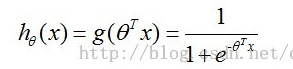]1 lr_2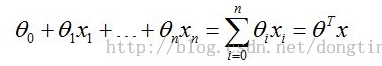]2 lr_1

h函数的原型函数为sigmoid函数，展示如下：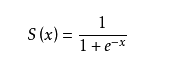]3 sigmoid_function

sigmoid方程的图形如下，sigmoid函数的取值范围为 (0,1)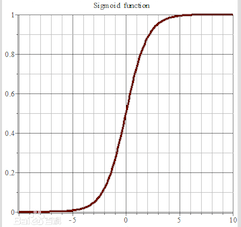]4 sigmoid_pic

#### j函数相关

j函数的目标就是找到一组最佳θ，使得J(θ)的值最小。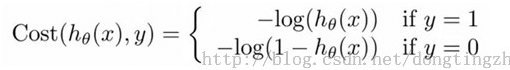]5 lr_4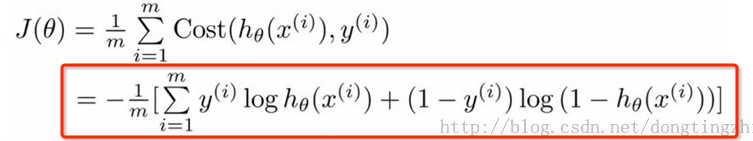]6 lr_5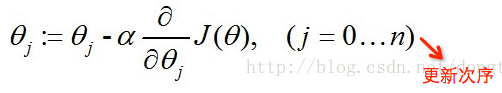]7 lr_6

ps：xj为x向量的第j分量，还可以理解为x数组的第j项，其实下图是对θ数组的第j项进行更新的算式，然而真正代码角度是对整个θ数组进行更新，也就是下下图的样子。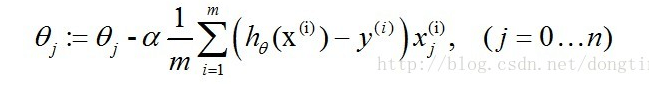]8 lr_7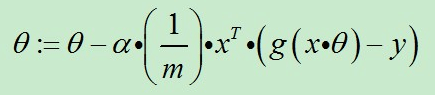]9 lr_8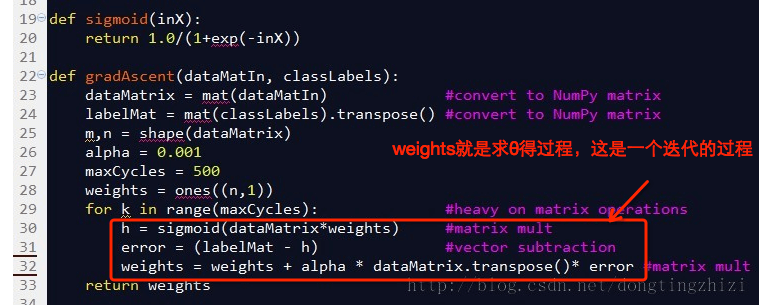]10 lr_9

### 逻辑回归的实际应用

```# !/usr/bin/env python

# -*- coding: utf-8 -*-

from sklearn import linear_model from sklearn.metrics import classification_report from sklearn.metrics import precision_recall_curve, roc_curve, auc

def main(): train_data = [] train_result = [] for i in open('train_data.txt').readlines(): ''' 29119 3.440948 0.078331 1 前三位为训练数据，最后一位为训练结果 ''' r = i[:-2].split('\t') train_data.append(r[:3]) train_result.append(r[-1])

clf = linear_model.LogisticRegression(max_iter=10000, C=1e5)
clf.fit(train_data, train_result)

print '输出预测结果'
print clf.predict([[68846, 9, 0.6]])

print '输出预测概率分布'
print clf.predict_proba([[68846, 9, 0.6]])

print 'decision function的系数'
print clf.coef_

print 'decision function的截距'
print clf.intercept_

```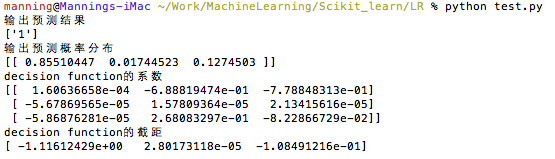]11 lr_10

### 逻辑回归的总结

Logistic Regression算法作为一个二分类算法，主要解决的是线性可分的问题，对于多分类算法，可以利用Softmax Regression算法。

Softmax Regression是一般化的Logistic Regression，可以把Logistic Regression看成Softmax Regression的特例。

Softmax 回归 vs. k 个二元分类器

### 参考内容：

http://ufldl.stanford.edu/wiki/index.php/Softmax%E5%9B%9E%E5%BD%92

http://blog.csdn.net/dongtingzhizi/article/details/15962797